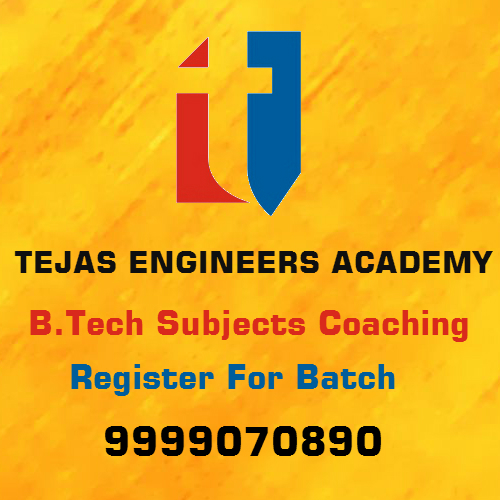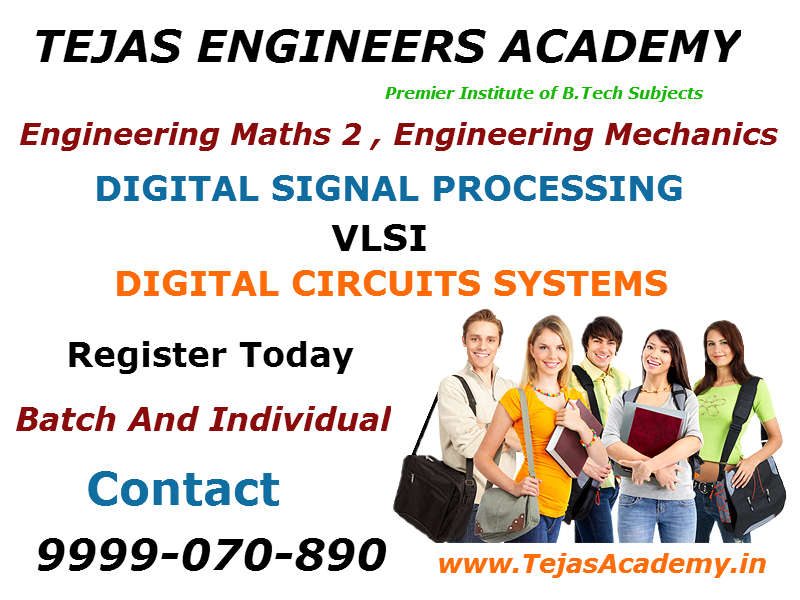# B.Tech Tutorials in Delhi tips for cracking DSP examIf you are a B.Tech student of Delhi studying in sixth semester then Digital signal processing will be a tough subject to clear. Following points are to be kept in mind to prepare first sessional examination of B.Tech tutorials in Delhi.

1.   First prepare notes for classification of signals. Most important is checking whether a signal is periodic or not. Also finding whether the signal is energy or power signal is also very important. Energy signal is that which has finite energy but zero power. Power signal is that which has finite power but infinite energy. Students generally get confused in self study and try to find B.Tech tutorials in Delhi.

2. Then prepare notes on various techniques on finding convolution. There are two types of convolutions : Linear convolution and circular convolution. Linear convolution involves linear shift operations whereas circular convolution involves circular shifting then superimposing the sequences. If you need B.Tech tutorials in Delhi for techniques of convolution then you can come to Tejas Engineers Academy for free classes.

3  Make notes on properties of systems. There are few properties of systems, such as linearity, Time invariance, Causal Non causal system, static and dynamic system, invertible and non invertible system, stable and unstable system. A system is said to be causal if its output depends upon present and previous values of system but not on future values. Checking whether the system is causal non causal, linear nonlinear are very important and every year a question comes in exam based on these topics. There are many institutes providing B.Tech tutorials in Delhi for properties of system.

4   Z-Transform is very important for first sessional exams. Z-Transform is a tool with which a give system can be converted from time domain to complex domain. When we take Z-Transform of system then it goes to complex domain and we can easily check whether the given system is stable or unstable by finding the location of its poles. If poles of a system lies inside the unit circle then it is stable otherwise it is unstable. Properties of z-Transform finding its Region of convergence is very important topic for which Tejas Engineers Academy provides Demo B.Tech tutorials in Delhi.